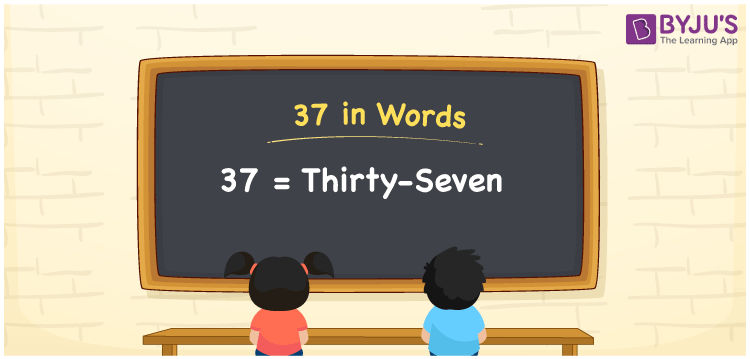# 37 in Words

37 in words can be written as Thirty-seven. The basic topics in Maths like counting can be learnt effectively in this article. If you buy a biscuit for Rs. 37, then you can say that “I bought a biscuit for Thirty-seven Rupees”. The English alphabet is made use of to write the numbers in words. The numbers in words topic is discussed here to enhance the foundation of basic concepts among students. 37 can be read as “Thirty-seven” in English.

 37 in words Thirty-seven Thirty-seven in Numbers 37

## 37 in English Words## How to Write 37 in Words?

Learn more about the place value chart and expanded form of 37. Two digits are present in 37. Using the place value chart, students will understand the basic concepts easily.

 Tens Ones 3 7

37 can be written in expanded form as:

3 × Ten + 7 × One

= 3 × 10 + 7 × 1

= 30 + 7

= 37

= Thirty-seven

Hence, 37 in words is written as Thirty-seven.

37 is a natural number that precedes 38 and succeeds 36.

37 in words – Thirty-seven

Is 37 an odd number? – Yes

Is 37 an even number? – No

Is 37 a perfect square number? – No

Is 37 a perfect cube number? – No

Is 37 a prime number? – Yes

Is 37 a composite number? – No

## Frequently Asked Questions on 37 in Words

Q1

### How to write 37 in words?

37 can be written in words as “Thirty-seven”.
Q2

### Write Thirty-seven in numbers.

Thirty-seven in numbers can be written as 37.
Q3

### Is 37 an odd or even number?

37 is an odd number as it is not completely divisible by 2.